# 1.7 R语言的高质量图形渲染库Cairo

#### 问题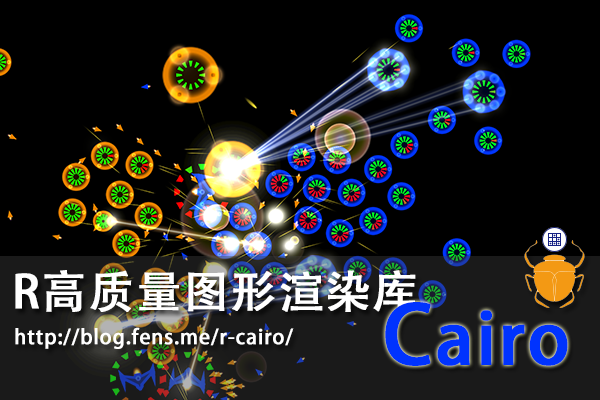#### 引言

R语言不仅在统计分析和数据挖掘领域计算能力强大，它在数据可视化领域也不逊于昂贵的商业软件。当然，R在可视化上强大，其背后离不开各种开源软件包的支持，Cairo就是这样一个用于矢量图形处理的类库。Cairo可以创建高质量的矢量图形(GIF, SVG, PDF, PostScript) 和位图(PNG, JPEG, TIFF)，同时支持在后台程序中高质量渲染！本节将介绍，Cairo在R语言中的使用。

## 1.7.1 Cairo介绍

Cairo 是一个用于图形绘图和渲染的免费库，支持复杂的 2D 的绘图功能，支持硬件加速。虽然，Cairo 是用C语言编写的，但提供多种语言的接口，允许其他语言直接调用，包括有 C++、C#、Java、Python、Perl、Ruby、Scheme、Smalltalk 等语言。Cairo发布的许可协议为 GNU Lesser General Public License version 2.1(LGPL) 或 Mozilla Public License 1.1(MPL)。R语言Cairo接口的官方发布页：http://www.rforge.net/Cairo/

## 1.7.2 Cairo包安装

• Linux: Ubuntu 12.04.2 LTS 64bit
• R: 3.0.1 x86_64-pc-linux-gnu

Cairo包在Linux Ubuntu系统中的安装过程如下：

``````~ sudo apt-get install libcairo2-dev  # Cairo的底层依赖库
~ sudo apt-get install libxt-dev
~ R  # 启动R程序
> install.packages("Cairo")  # 安装Cairo包
``````

## 1.7.3 Cairo使用

Cairo使用起来非常简单，和基础包grDevices中的函数对应。

• CairoPNG: 对应grDevices:png()
• CairoJPEG: 对应grDevices:jpeg()
• CairoTIFF: 对应grDevices:tiff()
• CairoSVG: 对应grDevices:svg()
• CairoPDF: 对应grDevices:pdf()

``````> library(Cairo)  # 加载Cairo包
> Cairo.capabilities()  # 检查Cairo包支持的图片格式
``````
png jpeg tiff pdf svg ps x11 win raster
TRUE TRUE FALSE TRUE TRUE TRUE TRUE FALSE TRUE

• 支持：png,jpeg,pdf,svg,ps,x11(Linux桌面),raster
• 不支持：tiff, win(win桌面)

### 1. 散点图

``````> x<-rnorm(6000)  # 随机取6000个点坐标
> y<-rnorm(6000)

> png(file="plot4.png",width=640,height=480)  # png函数
> plot(x,y,col="#ff000018",pch=19,cex=2,main = "plot")
> dev.off()

> CairoPNG(file="Cairo4.png",width=640,height=480)  # CairoPNG函数
> plot(x,y,col="#ff000018",pch=19,cex=2,main = "Cairo")
> dev.off()
``````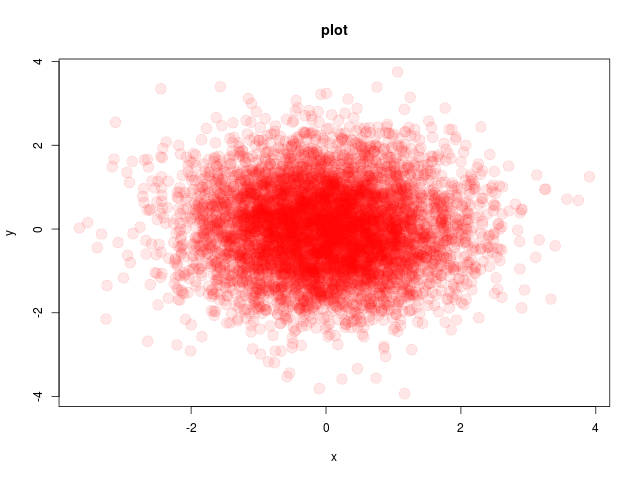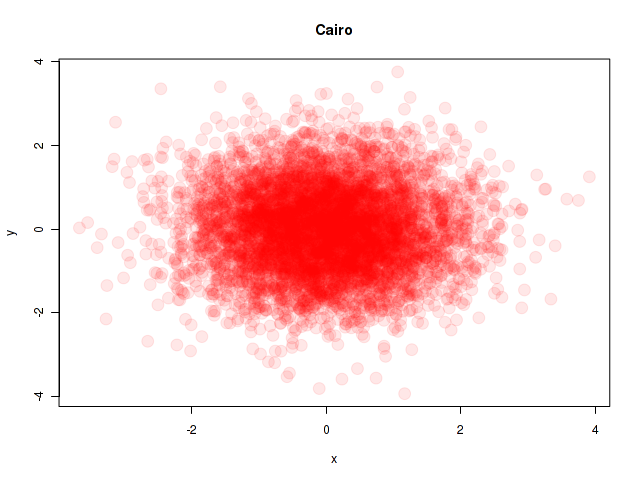SVG图形输出代码为：

``````> svg(file="plot-svg4.svg",width=6,height=6)
> plot(x,y,col="#ff000018",pch=19,cex=2,main = "plot-svg")
> dev.off()

> CairoSVG(file="Cairo-svg4.svg",width=6,height=6)
> plot(x,y,col="#ff000018",pch=19,cex=2,main = "Cairo-svg")
> dev.off()
``````

### 2. 三维截面图

``````> x <- seq(-10, 10, length= 30)
> y <- x
> f <- function(x,y) { r <- sqrt(x^2+y^2); 10 * sin(r)/r }
> z <- outer(x, y, f)
> z[is.na(z)] <- 1

> png(file="plot2.png",width=640,height=480)  # PNG图
> op <- par(bg = "white", mar=c(0,2,3,0)+.1)
> persp(x, y, z,theta = 30, phi = 30,expand = 0.5,col = "lightblue",ltheta = 120,shade = 0.75,ticktype = "detailed",xlab = "X", ylab = "Y", zlab = "Sinc(r)",main = "Plot")
> par(op)
> dev.off()

> CairoPNG(file="Cairo2.png",width=640,height=480)
> op <- par(bg = "white", mar=c(0,2,3,0)+.1)
> persp(x, y, z,theta = 30, phi = 30,expand = 0.5,col = "lightblue",ltheta = 120,shade = 0.75,ticktype = "detailed",xlab = "X", ylab = "Y", zlab = "Sinc(r)",main = "Cairo")
> par(op)
> dev.off()
``````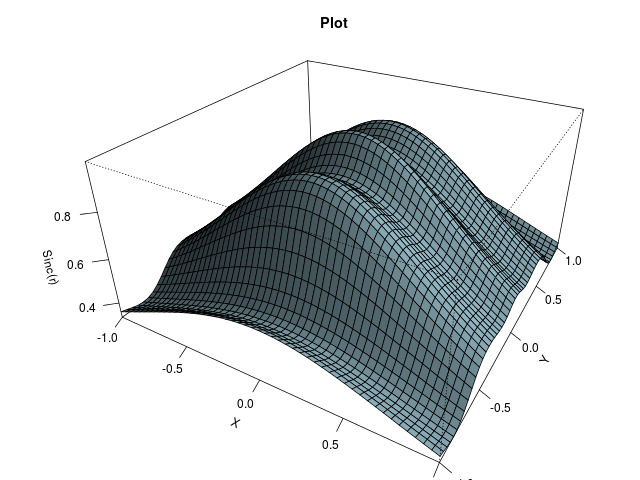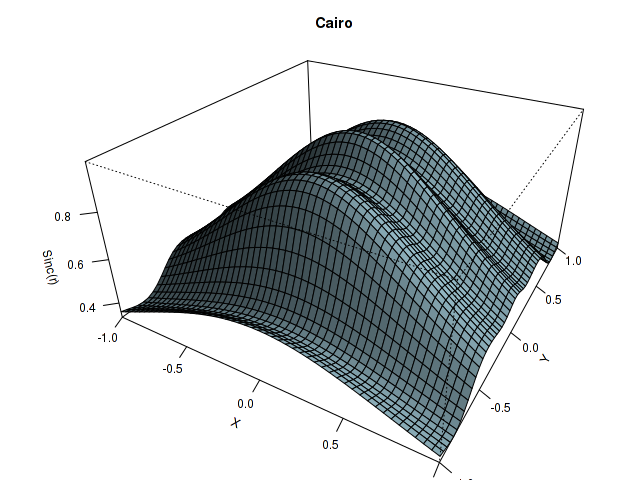### 3. 大量文字的图片

``````> library(MASS)  # 加载MASS包
> data(HairEyeColor)  # 加载HairEyeColor数据集
> x <- HairEyeColor[,,1]+HairEyeColor[,,2]
> n <- 100
> m <- matrix(sample(c(T,F),n^2,replace=T), nr=n, nc=n)
> png(file="plot5.png",width=640,height=480)  # PNG图
> biplot(corresp(m, nf=2), main="Plot")
> dev.off()

> CairoPNG(file="Cairo5.png",width=640,height=480)
> biplot(corresp(m, nf=2), main="Cairo")
> dev.off()
``````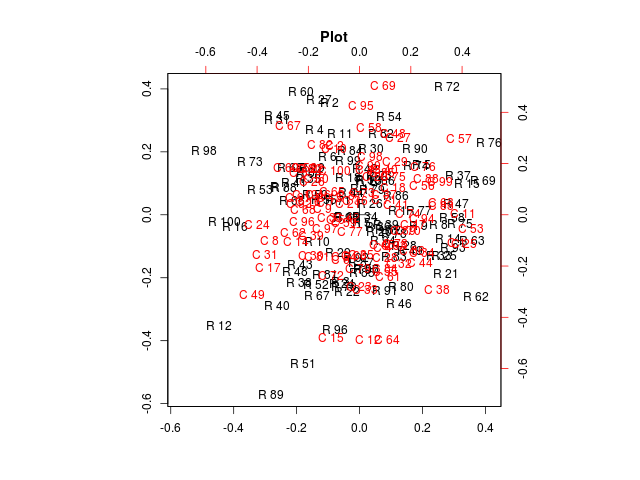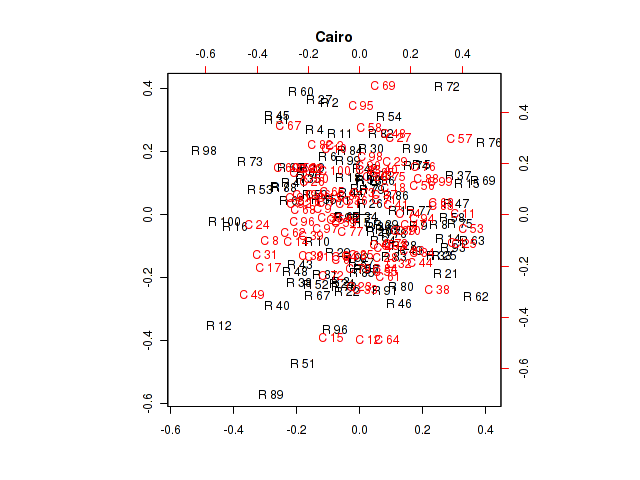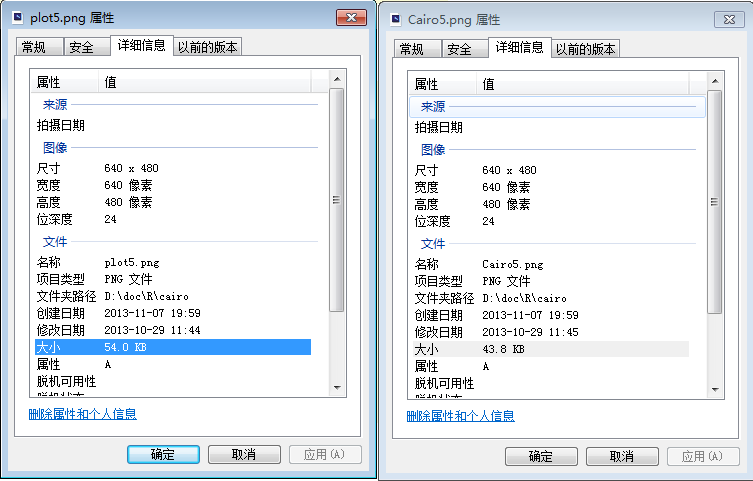SVG图形输出代码为：

``````> svg(file="plot-svg5.svg",width=6,height=6)
> biplot(corresp(m, nf=2), main="Plot-svg")
> dev.off()

> CairoSVG(file="Cairo-svg5.svg",width=6,height=6)
> biplot(corresp(m, nf=2), main="Cairo-svg")
> dev.off()
``````